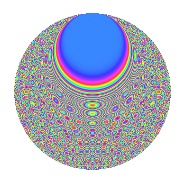# Properties

 Label 804.2.bf.bLevel 804 Weight 2 Character orbit 804.bf Analytic conductor 6.420 Analytic rank 0 Dimension 680 CM No

# Related objects

## Newspace parameters

 Level: $$N$$ = $$804 = 2^{2} \cdot 3 \cdot 67$$ Weight: $$k$$ = $$2$$ Character orbit: $$[\chi]$$ = 804.bf (of order $$66$$ and degree $$20$$)

## Newform invariants

 Self dual: No Analytic conductor: $$6.41997232251$$ Analytic rank: $$0$$ Dimension: $$680$$ Relative dimension: $$34$$ over $$\Q(\zeta_{66})$$ Sato-Tate group: $\mathrm{SU}(2)[C_{66}]$

## $q$-expansion

The dimension is sufficiently large that we do not compute an algebraic $$q$$-expansion, but we have computed the trace expansion.

 $$\operatorname{Tr}(f)(q) =$$ $$680q + 68q^{3} + 2q^{4} + 11q^{6} - 4q^{7} - 27q^{8} - 68q^{9} + O(q^{10})$$ $$\operatorname{Tr}(f)(q) =$$ $$680q + 68q^{3} + 2q^{4} + 11q^{6} - 4q^{7} - 27q^{8} - 68q^{9} + 15q^{10} + 9q^{12} - 6q^{13} - 10q^{14} + 2q^{16} + 36q^{20} + 4q^{21} + 33q^{22} - 6q^{24} + 68q^{25} + q^{26} + 68q^{27} + 76q^{28} + 8q^{29} + 18q^{30} - 2q^{31} + 40q^{32} - 9q^{36} - 12q^{37} + 22q^{38} + 6q^{39} + 37q^{40} + 10q^{42} + 4q^{43} - 123q^{44} + 67q^{46} - 2q^{48} + 46q^{49} + 42q^{50} + 28q^{52} + 11q^{56} - 66q^{57} - 92q^{58} + 74q^{60} - 6q^{61} + 34q^{62} + 18q^{63} - 49q^{64} - 22q^{66} + 18q^{67} + 208q^{68} - 56q^{70} + 36q^{71} + 6q^{72} - 72q^{73} + 53q^{74} - 68q^{75} - 190q^{76} + 4q^{77} - q^{78} - 28q^{79} + 11q^{80} - 68q^{81} - 84q^{82} - 12q^{83} + q^{84} - 89q^{86} - 8q^{87} + 160q^{88} + 15q^{90} + 166q^{92} + 2q^{93} + 16q^{94} - 20q^{95} + 15q^{96} - 18q^{97} + 37q^{98} + O(q^{100})$$

## Embeddings

For each embedding $$\iota_m$$ of the coefficient field, the values $$\iota_m(a_n)$$ are shown below.

For more information on an embedded modular form you can click on its label.

Label $$a_{2}$$ $$a_{3}$$ $$a_{4}$$ $$a_{5}$$ $$a_{6}$$ $$a_{7}$$ $$a_{8}$$ $$a_{9}$$ $$a_{10}$$
7.1 −1.41418 + 0.00970285i 0.959493 + 0.281733i 1.99981 0.0274432i −0.723786 0.627165i −1.35963 0.389111i 0.163159 3.42512i −2.82783 + 0.0582134i 0.841254 + 0.540641i 1.02965 + 0.879901i
7.2 −1.41249 + 0.0698158i 0.959493 + 0.281733i 1.99025 0.197228i −2.97198 2.57523i −1.37494 0.330956i −0.164722 + 3.45794i −2.79744 + 0.417533i 0.841254 + 0.540641i 4.37768 + 3.43000i
7.3 −1.39654 + 0.222851i 0.959493 + 0.281733i 1.90067 0.622444i 0.851506 + 0.737834i −1.40276 0.179628i −0.200085 + 4.20030i −2.51566 + 1.29284i 0.841254 + 0.540641i −1.35359 0.840659i
7.4 −1.35999 0.387841i 0.959493 + 0.281733i 1.69916 + 1.05492i 1.14805 + 0.994791i −1.19564 0.755285i 0.0104266 0.218882i −1.90170 2.09369i 0.841254 + 0.540641i −1.17552 1.79817i
7.5 −1.32902 + 0.483424i 0.959493 + 0.281733i 1.53260 1.28496i 0.0351858 + 0.0304887i −1.41138 + 0.0894134i 0.111602 2.34282i −1.41568 + 2.44864i 0.841254 + 0.540641i −0.0615018 0.0235105i
7.6 −1.28701 0.586177i 0.959493 + 0.281733i 1.31279 + 1.50883i 1.87962 + 1.62870i −1.06973 0.925026i −0.129508 + 2.71872i −0.805133 2.71141i 0.841254 + 0.540641i −1.46439 3.19795i
7.7 −1.22292 0.710261i 0.959493 + 0.281733i 0.991060 + 1.73718i −2.24944 1.94915i −0.973278 1.02603i 0.0649402 1.36326i 0.0218664 2.82834i 0.841254 + 0.540641i 1.36648 + 3.98134i
7.8 −1.08084 + 0.912026i 0.959493 + 0.281733i 0.336415 1.97150i 2.38928 + 2.07032i −1.29400 + 0.570576i 0.134687 2.82743i 1.43445 + 2.43769i 0.841254 + 0.540641i −4.47061 0.0585939i
7.9 −0.959598 1.03883i 0.959493 + 0.281733i −0.158342 + 1.99372i −0.243036 0.210592i −0.628055 1.26710i 0.0786161 1.65036i 2.22309 1.74868i 0.841254 + 0.540641i 0.0144475 + 0.454558i
7.10 −0.944404 + 1.05266i 0.959493 + 0.281733i −0.216203 1.98828i −1.97935 1.71512i −1.20272 + 0.743954i −0.0520043 + 1.09170i 2.29717 + 1.65015i 0.841254 + 0.540641i 3.67475 0.463828i
7.11 −0.893496 + 1.09620i 0.959493 + 0.281733i −0.403329 1.95891i 1.84848 + 1.60172i −1.16614 + 0.800074i −0.0574419 + 1.20585i 2.50774 + 1.30815i 0.841254 + 0.540641i −3.40743 + 0.595185i
7.12 −0.745475 1.20178i 0.959493 + 0.281733i −0.888534 + 1.79179i 3.24307 + 2.81014i −0.376699 1.36312i −0.0543857 + 1.14170i 2.81571 0.267916i 0.841254 + 0.540641i 0.959528 5.99234i
7.13 −0.608186 1.27676i 0.959493 + 0.281733i −1.26022 + 1.55301i −1.21985 1.05701i −0.223846 1.39639i −0.201271 + 4.22520i 2.74927 + 0.664476i 0.841254 + 0.540641i −0.607646 + 2.20031i
7.14 −0.484970 + 1.32846i 0.959493 + 0.281733i −1.52961 1.28853i 0.614520 + 0.532485i −0.839596 + 1.13802i −0.193124 + 4.05416i 2.45357 1.40713i 0.841254 + 0.540641i −1.00541 + 0.558126i
7.15 −0.278535 1.38651i 0.959493 + 0.281733i −1.84484 + 0.772385i 0.342563 + 0.296833i 0.123373 1.40882i −0.0123453 + 0.259160i 1.58477 + 2.34275i 0.841254 + 0.540641i 0.316146 0.557646i
7.16 −0.0734396 + 1.41231i 0.959493 + 0.281733i −1.98921 0.207438i −1.96466 1.70238i −0.468357 + 1.33441i −0.0298864 + 0.627393i 0.439053 2.79414i 0.841254 + 0.540641i 2.54857 2.64967i
7.17 −7.50135e−5 1.41421i 0.959493 + 0.281733i −2.00000 0.000212170i −1.53250 1.32792i 0.398358 1.35695i 0.182174 3.82430i 0.000450081 2.82843i 0.841254 + 0.540641i −1.87785 + 2.16738i
7.18 0.112466 + 1.40973i 0.959493 + 0.281733i −1.97470 + 0.317094i 1.50481 + 1.30392i −0.289258 + 1.38432i 0.198031 4.15718i −0.669104 2.74814i 0.841254 + 0.540641i −1.66894 + 2.26802i
7.19 0.196375 + 1.40051i 0.959493 + 0.281733i −1.92287 + 0.550052i −1.67222 1.44899i −0.206150 + 1.39911i −0.0358317 + 0.752200i −1.14796 2.58499i 0.841254 + 0.540641i 1.70094 2.62651i
7.20 0.319658 1.37761i 0.959493 + 0.281733i −1.79564 0.880730i 2.42058 + 2.09744i 0.694828 1.23175i 0.217948 4.57528i −1.78730 + 2.19216i 0.841254 + 0.540641i 3.66322 2.66415i
See next 80 embeddings (of 680 total)
 $$n$$: e.g. 2-40 or 990-1000 Embeddings: e.g. 1-3 or 787.34 Significant digits: Format: Complex embeddings Normalized embeddings Satake parameters Satake angles

## Inner twists

This newform does not have CM; other inner twists have not been computed.

## Hecke kernels

This newform can be constructed as the kernel of the linear operator $$T_{7}^{680} + \cdots$$ acting on $$S_{2}^{\mathrm{new}}(804, [\chi])$$.